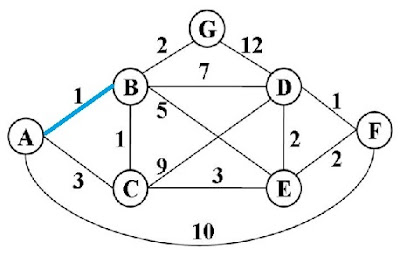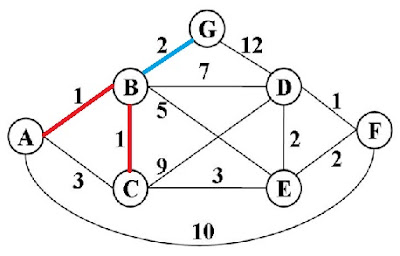Please visit, subscribe and share 10 Minutes Lectures in Computer Science

# Dijkstra's algorithm in data structures, single source shortest path algorithm solved exercise

Question: Consider the given undirected weighted graph. Use Dijkstra’s algorithm to calculate the single-source shortest paths from A to every other vertex.

Solution:

 Vertex Known Cost from source A Minimum cost to reach next vertex Next vertex chosen Visited vertices A (source) Y 0 - A From A, we can go to either B, or C, or F B Y 1 Choose next vertex as follows; minimum(AB, AC, AF) = min(1, 3, 10) = 1 Choose B (cost = 1) as the next vertex. B AB C Y 3 F Y 10 Vertices visitedFrom B, we can go to C, D, E, or G and from A we can reach C directly C Y AB+BC = 1+1 = 2 Min(ABC, ABD, ABE, ABG, AC) = min(2, 8, 6, 3, 3) = 2 Choose C (cost = 2) as the next vertex. C ABC D Y AB+BD = 1+7 = 8 E Y AB+BE = 1+5 = 6 G Y AB + BG = 1+2 = 3 C Y AC = 3 Vertices visitedFrom C, we can reach D or E and from B we can reach G D Y ABC+CD = 2+9 = 11 Min(ABCD, ABCE, ABG) = min(11, 5, 3) = 3 Choose G (cost = 3) as the next vertex. G ABCG E Y ABC+CE = 2+3 = 5 G Y AB+BG = 1+2 = 3 Vertices visitedFrom G, we can go to D and from C we can go to D or E D Y ABG+GD = 3+12 = 15 Min(ABGD, ABCE, ABCD) = min(15, 5, 11) = 5 Choose E (cost = 5) as the next vertex. E ABCEG E Y ABC+CE = 2+3 = 5 D Y ABC+CD = 2+9 = 11 Vertices visitedNext we can reach D or F D Y ABG+GD = 3+12 = 15 Min(ABGD, ABD, ABCED, ABCD, ABCEF, AF) = min(15, 8, 7, 11, 7, 10) = 7   Choose D or F (cost = 7) as the next vertex.   After D, we can choose F (cost = 7) as the next vertex. D F ABCDEFG D Y AB+BD = 1+7 = 8 D Y ABCE+ED = 5+2 = 7 D Y ABC+CD = 2+9 = 11 F Y ABCE+EF = 5+2 = 7 F Y AF = 10

The shortest path will be;

From this graph, we can get the shortest path from single source (A in this case) to any other vertices. For instance, the shortest path from A to D is ABCED with the cost 7 (AB+BC+CE+ED). The shortest path from A to G is ABG with the cost 3 (AB+BG). The shortest path from A to C is ABC with the cost 2 (AB+BC) etc.

****************

## Featured Content

### Multiple choice questions in Natural Language Processing Home

MCQ in Natural Language Processing, Quiz questions with answers in NLP, Top interview questions in NLP with answers Multiple Choice Que...

data recovery